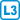## Crossing chords

In a convex $$n$$-gon, how many pairs of chords exist that intersect inside the $$n$$-gon, i.e. not at boundary points?

• #### Solution

Every pair of chords gives us a quadruple of vertices, the endpoints of the chords.

Conversely, if we choose a quadruple of vertices, we can then choose only one pair of chords that will intersect. (With the other two possibilities, the chords do not intersect.)

The number of pairs of chords is $$\binom{n}{4}$$.